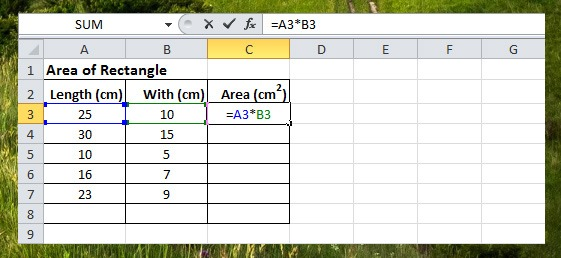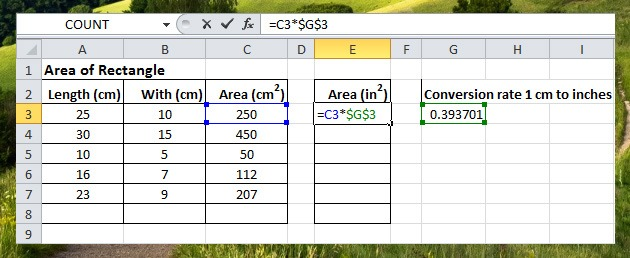# Cell Reference

A worksheet also known as the spreadsheet is composed of a box-like structure called a cell. Every cell has an address location called cell reference. A cell reference consists of a column letter and row number (e.g. A1, D63, F120), this is where the row and column intersect.

Cell reference is used in Excel commands like formulas, functions and charts. In this lesson you will understand the three types of cell references, Relative, Absolute and Mixed. Let’s take a look one by one.

Relative Cell Reference

Relative reference means that when you change the location the cell address also changes or the cell reference is relative to its location, by default Excel uses relative reference.

Take a look at the example below, cell C3 formula points to cell A3 and B3, cell references are relative.Now if you drag down and copy the formula in cell C3 up to C7 the cell reference also change. C4 references cell A4 and B4, C5 references cell A5 and B5 and so on. This means that cell C3 up to C7 are relative to the two cells on the left.Absolute Cell Reference

Absolute reference means the cell reference will be fixed to it’s location address when you move or copy the cell to any location. This can be done by adding a \$ symbol in front of the column letter and row number.

Take a look at the example below in cell E3, we put a \$ symbol on a reference cell G3 (conversion rate) to make it absolute.So when we drag the formula down to E7, the G3 cell reference will not move.Mixed Cell Reference

Mixed reference is the combination of relative and absolute, in the example below reference column B which is the price and reference row 3 the % off price are fixed. So when we drag the formula in Cell F4 to the right, the price reference will be fixed and the % Off price reference will be relative.When we drag the formula down, the % Off price reference will be fixed and the price reference will be relative.#### One Response

1.Catherine